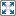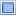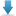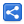# TypeScript - Using Interfaces to describe Functions

[Last Updated: Sep 6, 2018]

In TypeScript, interfaces can also describe functions. In other words interfaces can be defined as reusable types for function. We just need to define function signature in the interface. Let's understand that with an example.

## Example

In following example, we are writing an ordinary function in TypeScript:

#### without-describing-function.ts

```function removeByIndex(str: string, i: number): string{
return str.substring(i);
}

let s = removeByIndex("Hi there", 3);
console.log(s);```

#### Output

`there`

Now let's create an interface describing above function:

#### describing-function.ts

```//reusable type for function
interface StringRemover {
//describing function,
//parameters are in left side parenthesis,
//right side 'string' is return type
(input: string, index: number): string;
}

let remover: StringRemover = function (str: string, i: number): string {
return str.substring(i);
}

let s = remover("Hi there", 3);
console.log(s);
```

#### Output

`there`

As seen above, the parameter names should not necessarily be the same when writing a typed function, but their types should be in the same order.

Following is the compiled JavaScript code of above example:

#### describing-function.js

```let remover = function (str, i) {
return str.substring(i);
};
let s = remover("Hi there", 3);
console.log(s);
```

Just like interface describing object properties, the advantage of interface describing a function is compile time strong typing. If we violate any typing rules on function definition then compiler will warn us.

In following example we are using a different type than the one described in the interface:

#### describing-function2.ts

```interface StringRemover {
(input: string, index: number): string;
}

let remover: StringRemover = function (a: number, b: number): number {
return a + b;
}

```

#### Output

`describing-function2.ts(5,5): error TS2322: Type '(a: number, b: number) => number' is not assignable to type 'StringRemover'.  Types of parameters 'a' and 'input' are incompatible.    Type 'string' is not assignable to type 'number'.`

## Example Project

Dependencies and Technologies Used:

• TypeScript 3.0.1
 Using interfaces to describe FunctionsSelect AllDownload• typescript-interface-describing-function
• describing-function.ts

 Share